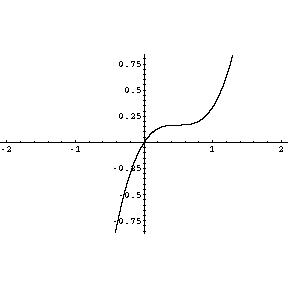Quandaries and Queries Hello, I was wondering how do you figure out if a graph has a horizontal tangent line. One of my homework problem was to sketch the graph of the following function; (4/3)x3-2x2+x. I set f''(x) ( the second derivative) of the function equal to zero and got the inflection point:(1/2,1/6). Also i am having trouble finding the concavity for x>1/2 and x<1/2, i am getting a different answer from the back of the book, the graph i draw looks completely different from the correct answer. Hi, There is a horizontal tangent line at a point on the graph where the first derivative is zero. In this example f'(x) = 4x2 - 4x + 1 = (2x - 1)2 and hence there is a horizontal tangent at x = 1/2. Also the first derivative is a square and hence never negative, which implies that the curve is increasing. The graph that I get isPenny Go to Math Central